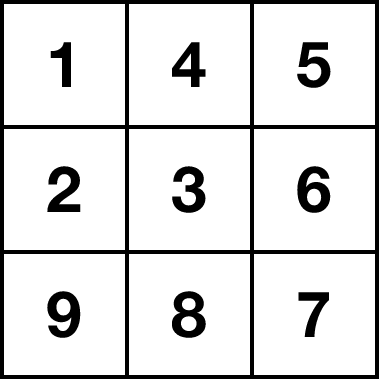# A beautiful problem from a Hungarian competition

Probability Level 4

We need to fill each cell in a $6\times6$ grid with a distinct integer from 1 to 36. There are two rules:

• Every pair of consecutive numbers are in adjacent cells that share an edge.
• Any two cells containing a multiple of 4 cannot share an edge nor a vertex.

Over all valid configurations, how many of the 36 cells could contain the number $36?$


Note: Below is a $3\times 3$ grid adhering to the rules above.×